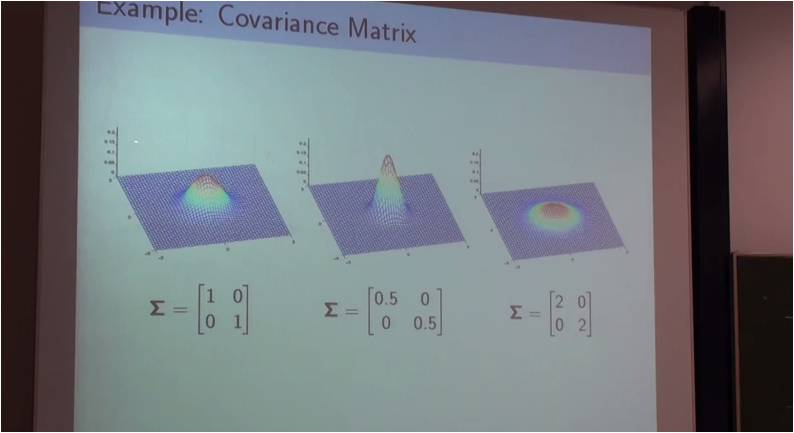# InfoCoBuild

## Advanced Mathematics for Engineers 2

Advanced Mathematics for Engineers 2 (Hochschule Ravensburg-Weingarten Univ.). Instructor: Professor Wolfgang Ertel. After a repetition of basic linear algebra, computer algebra and calculus, this course will deal with numerical calculus, statistics and function approximation, which are the most important basic mathematics topics for engineers in the fields of computer science, mechatronics and electrical engineering.

Topics covered in this course, Advanced Mathematics for Engineers 2, include: random numbers including Kolmogorov complexity and pseudo random number generators; statistics and probability including principal component analysis, estimators, the Gaussian distribution, and linear regression; function approximation: clustering, k-Means and the EM-Algorithm, and singular value decomposition; numerical integration and solution of ordinary differential equations.Lecture 01 Lecture 02 - Random Numbers: Kolmogorov Complexity, Pseudo Random Number Generators Lecture 03 - The Symmetry Test, BBS Generator (Blum Blum Shub) Lecture 04 - True Random Numbers, The Neumann Filter Lecture 05 - Linear Feedback Shift Registers, Derivation of a suitable Speedup Formula Lecture 06 - Statistics and Probability: Principal Component Analysis (PCA) Lecture 07 - Principal Component Analysis (PCA), Estimators, The Gaussian Distribution Lecture 08 - Maximum Likelihood, Linear Regression Lecture 09 - Applications of PCA (An Image Compression Example) Lecture 10 - Maximum Likelihood, Bayesian Linear Regression, Radial Basis Functions (RBFs) Lecture 11 - Demonstration of Galton Machine: Octave Examples Lecture 12 - Function Approximation: Clustering, k-Means and the EM-Algorithm, Singular Value Decomposition Lecture 13 - Singular Value Decomposition Lecture 14 - Numerical Integration and Solution of Ordinary Differential Equations Lecture 15 - Numerical Integration, Numerical Differentiation Lecture 16 - Numerical Solution of Ordinary Differential Equations Lecture 17 - Numerical Solution of Ordinary Differential Equations, Linear Differential Equations Lecture 18 - Linear Differential Equations with Constant Coefficients

 References Advanced Mathematics for Engineers | Hochschule Ravensburg-Weingarten Instructor: Professor Wolfgang Ertel. Demos and Course Material. Octave Exam Programs. Previous Examinations. Video Lectures from Previous Semesters.